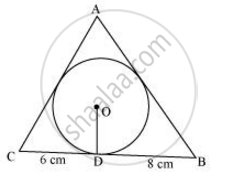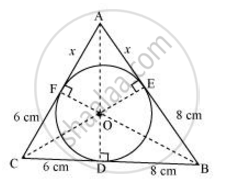# A triangle ABC is drawn to circumscribe a circle of radius 4 cm such that the segments BD and DC into which BC is divided by the point of contact D are of lengths 8 cm and 6 cm respectively - Mathematics

A triangle ABC is drawn to circumscribe a circle of radius 4 cm such that the segments BD and DC into which BC is divided by the point of contact D are of lengths 8 cm and 6 cm respectively (see given figure). Find the sides AB and AC.#### SolutionLet the given circle touch the sides AB and AC of the triangle at point E and F respectively and the length of the line segment AF be x.

In ΔABC,

CF = CD = 6cm (Tangents on the circle from point C)

BE = BD = 8cm (Tangents on the circle from point B)

AE = AF = x (Tangents on the circle from point A)

AB = AE + EB = x + 8

BC = BD + DC = 8 + 6 = 14

CA = CF + FA = 6 + x

2s = AB + BC + CA

x + 8 + 14 + 6 + x

= 28 + 2x

s = 14 + x

Area of triangleABC = sqrt(s(s-1)(s-b)(s-c))

=sqrt({14+x}{(14+x)-14}{(14+x)-(6+x)}{(14+x)-(8+x)})

=sqrt((14+x)(x)(8)(6))

=4sqrt(3(14x+x^2))

Area of triangleOBC = 1/2xxODxxBC = 1/2xx4xx14=28

Area of triangleOCA = 1/2xxOFxxAC = 1/2xx4xx(6+x)=12+2x

Area of triangleOAB = 1/2xxOExxAB = 1/2xx4xx(8+x)=16+2x

Area of ΔABC = Area of ΔOBC + Area of ΔOCA + Area of ΔOAB

4sqrt(3(14x+x^2)) = 28+12+2x+16+2x

4sqrt(3(14x+x^2)) = 56+4x

=>sqrt(3(14x+x^2)) = 14+x

=>3(14x+x^2) = (14+x)^2

=>42x+3x^2=196+x^2+28x

=>2x^2+14x-196 = 0

=>x^2+7x-98 = 0

=>x^2+14x-7x-98=0

=>x(x+14)-7(x+14) =0

=>(x+14)(x-7) =0

Either x+14 = 0 or x − 7 =0

Therefore, x = −14and 7

However, = −14 is not possible as the length of the sides will be negative.

Therefore, x = 7

Hence, AB = x + 8 = 7 + 8 = 15 cm

CA = 6 + x = 6 + 7 = 13 cm

Concept: Number of Tangents from a Point on a Circle
Is there an error in this question or solution?

#### APPEARS IN

NCERT Class 10 Maths
Chapter 10 Circles
Exercise 10.2 | Q 12 | Page 214